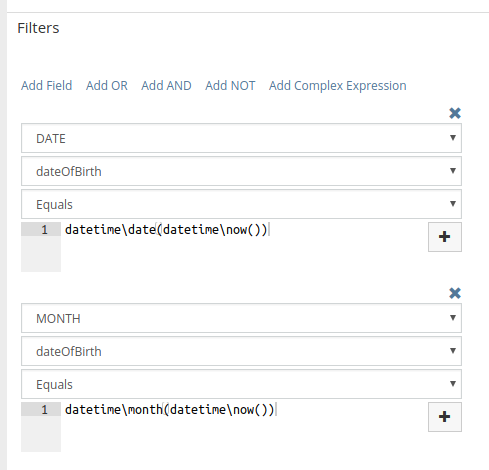# Report showing contacts that have birthday

Note: This feature is available since EspoCRM 4.7.0 and Advanced Pack 1.22.0.

Assume that we have the field dateOfBirth in Contact entity.

1. Create new list report for Contacts.
2. In the filter section click Add Complex Expression and specify fields like on the screenshot.Formula expression datetime\date(datetime\now()) gives the day number of the current month. Function DATE applied to dateOfBirth field gives the number of the month of dateOfBirth.

Formula expression datetime\month(datetime\now()) gives the current month number. Function MONTH applied to dateOfBirth field gives the month number of dateOfBirth.

It’s also possible to make up other expressions.

Birthday tomorrow:

``` DATE dateOfBirth Equals datetime\date(datetime\addDays(datetime\now(), 1))```

``` ```

```MONTH dateOfBirth Equals datetime\month(datetime\addDays(datetime\now(), 1)) ```

Birthday the next month:
``` MONTH dateOfBirth Equals datetime\month(datetime\addMonths(datetime\now(), 1)) ```